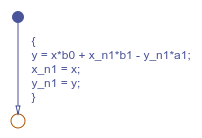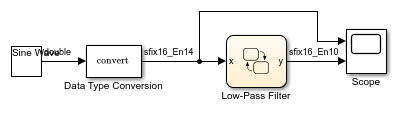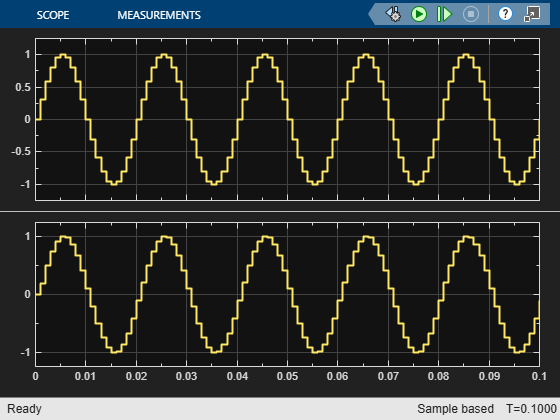# Build a Low-Pass Filter by Using Fixed-Point Data

This example shows how to build a Stateflow® chart that uses fixed-point data to implement a low-pass Butterworth filter. By designing the filter with fixed-point data instead of floating-point data, you can simulate your model using less memory. For more information, see Fixed-Point Data in Stateflow Charts.### Build the Fixed-Point Butterworth Filter

The Low-Pass Filter chart is a stateless flow chart that accepts one input and provides one output. The chart contains these data symbols:

• `x`Scope: `Input`, Type: `Inherit:Same as Simulink`

• `y`Scope: `Output`, Type: `fixdt(1,16,10)`

• `x_n1`Scope: `Local`, Type: `fixdt(1,16,12)`

• `y_n1`Scope: `Local`, Type: `fixdt(1,16,10)`

• `b0`Scope: `Parameter`, Type: `fixdt(1,16,15)`

• `b1`Scope: `Parameter`, Type: `fixdt(1,16,15)`

• `a1`Scope: `Parameter`, Type: `fixdt(1,16,15)`

The values of `b0`, `b1`, and `a1` are the coefficients of the low-pass Butterworth filter.

To build the Low-Pass Filter chart:

1. Create a Simulink® model with an empty Stateflow chart by entering `sfnew` at the MATLAB® command prompt.

2. In the Stateflow chart, add a flow chart with a single branch that assigns values to `y`, `x_n1`, and `y_n1`.

3. Add input, output, local, and parameter data to the chart, as described in Add Stateflow Data.

### Define the Model Callback Function

Before loading the model, MATLAB calls the `butter` (Signal Processing Toolbox) function to compute the values for the parameters `b0`, `b1`, and `a1`. The function constructs a first-order low-pass Butterworth filter with a normalized cutoff frequency of `(2*pi*Fc/(Fs/2))` radians per second, where:

• The sampling frequency is `Fs` = 1000 Hz.

• The cutoff frequency is `Fc` = 50 Hz.

The function output `B` contains the numerator coefficients of the filter in descending powers of `z`. The function output `A` contains the denominator coefficients of the filter in descending powers of `z`.

```Fs = 1000; Fc = 50; [B,A] = butter(1,2*pi*Fc/(Fs/2)); b0 = B(1); b1 = B(2); a1 = A(2); ```

To define the preload callback for the model:

1. In the Modeling tab, under Setup, select Model Settings > Model Properties.

2. In the Model Properties dialog box, on the Callbacks tab, select PreLoadFcn.

3. Enter the MATLAB code for the preload function call.

4. Click OK.

To load the parameter values to the MATLAB workspace, save, close, and reopen the model.

### Add Other Blocks to the Model

To complete the model, add a Sine Wave (Simulink) block, a Data Type Conversion (Simulink) block, and a Scope (Simulink) block. Connect and label the blocks according to this diagram.Sine Wave block

The Sine Wave block outputs a floating-point signal. The block has these settings:

• Sine type: `Time based`

• Time: `Use simulation time`

• Amplitude: `1`

• Bias: `0`

• Frequency: `2*pi*Fc`

• Phase: `0`

• Sample time: `1/Fs`

• Interpret vector parameters as 1-D: `On`

Data Type Conversion block

The Data Type Conversion block converts the floating-point signal from the Sine Wave block to a fixed-point signal. By converting the signal to a fixed-point type, you can simulate your model using less memory. The block has these settings:

• Output minimum: `[]`

• Output maximum: `[]`

• Output data type: `fixdt(1,16,14)`

• Lock output data type setting against changes by the fixed-point tools: `Off`

• Input and output to have equal: `Real World Value (RWV)`

• Integer rounding mode: `Floor`

• Saturate on integer overflow: `Off`

• Sample time: `-1`

Scope block

The Scope block has two input ports that connect to the input and output signals for the Low-Pass Filter chart. To display the two signals separately, select a scope layout with two rows and one column.

### Set Model Configuration Parameters

Because none of the blocks in the model have a continuous sample time, use a discrete solver with these configuration parameters:

• Stop time: `0.1`

• Type: `Fixed-step`

• Solver: `discrete (no continuous states)`

• Fixed-step size (fundamental sample time): `1/Fs`

To configure the model:

1. In the Modeling tab, under Setup, select Model Settings.

2. In the Solver pane, set the discrete solver parameters.

3. Click OK.

### Run the Model

When you simulate the model, the Scope block displays two signals. The top signal shows the fixed-point version of the sine wave input to the chart. The bottom signal corresponds to the filtered output from the chart. The filter removes high-frequency values from the signal but allows low-frequency values to pass through the chart unchanged.